Courses

# Revisal Problems (Past 13 Year) JEE Main (P Block Elements)

## 30 Questions MCQ Test Chemistry for JEE Advanced | Revisal Problems (Past 13 Year) JEE Main (P Block Elements)

Description
This mock test of Revisal Problems (Past 13 Year) JEE Main (P Block Elements) for JEE helps you for every JEE entrance exam. This contains 30 Multiple Choice Questions for JEE Revisal Problems (Past 13 Year) JEE Main (P Block Elements) (mcq) to study with solutions a complete question bank. The solved questions answers in this Revisal Problems (Past 13 Year) JEE Main (P Block Elements) quiz give you a good mix of easy questions and tough questions. JEE students definitely take this Revisal Problems (Past 13 Year) JEE Main (P Block Elements) exercise for a better result in the exam. You can find other Revisal Problems (Past 13 Year) JEE Main (P Block Elements) extra questions, long questions & short questions for JEE on EduRev as well by searching above.
QUESTION: 1

### Direction (Q. Nos. 1-30) This section contains 30 multiple choice questions. Each question has four choices (a), (b), (c) and (d), out of which ONLY ONE is correct. Q.  The true statement for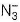is

Solution:

The azide ion () has 16 electrons (five from each N and one from the charge on the ion). It is isoelectronic with N2O.
It has linear structure called pseudo halide ion not pseudo halogen

QUESTION: 2

### One mole of each H3PO2, H3PO3 and H3PO4 will neutralise x moles of NaOH. y moles of Ca(OH)2 and z moles of AI(OH)3. Here x, y, z ratio is

Solution:

H3PO2, H3PO3 and H3PO4 are monobasic, dibasic and tribasic acids respectively.

QUESTION: 3

### When heated which metal nitrate would give the respective metal, oxide NO2 and O2?

Solution: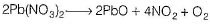QUESTION: 4

Which shape correctly describes the stated molecule?

Solution:

NF3 is sp3-hybridised and have trigonal pyramidal shape.

QUESTION: 5

P4O10 in cold and hot water produce respectively

Solution:

It readily dissolves in cold water forming metaphosphoric acid.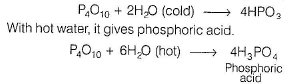QUESTION: 6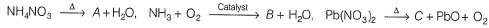Among A,B,C, paramagnetic substance(s) is/are

Solution: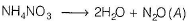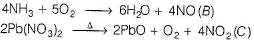Both nitric oxide (NO) and nitrogen dioxide (NO2) are paramagnetic in nature due to the presence of odd number of electron(s) in outer shell.

QUESTION: 7

Ammonium dichromate on heating 'X’ gas is liberated. The same gas is liberated by heating

Solution: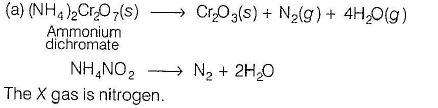QUESTION: 8

Which has more covalent bonds?

Solution: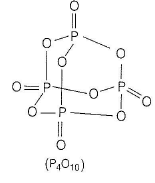QUESTION: 9

When sulphuric acid reacts with PCI5, sulphuryl chloride is formed as the final product. This shows that sulphuric acid is

Solution: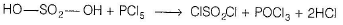QUESTION: 10

S—S bond is present in

I. H2S2O6
II. H2S2O8
III. H2S2O7
IV. H2 S2 O5

Solution: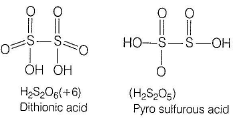QUESTION: 11

Which one of the following cannot act as an oxidising agent?

Solution:

In this sulphur has lowest oxidation state. It acts as reducing agent not oxidising agent.

QUESTION: 12

The V -shape of SO2 is due to the presence

Solution: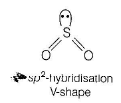QUESTION: 13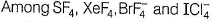the number of species having two lone pairs of electrons on the central atom according to VSEPR theory is

Solution: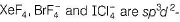hybridised with square planar shape.

QUESTION: 14

Liquid oxygen and liquid nitrogen are allowed to flow between the poles of an electromagnet. Choose the correct observation

Solution:

In O2 molecule, the antibonding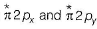orbitals are singly occupied. Hence, it is paramagnetic in nature.

QUESTION: 15

Among the species,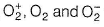the order of first ionisation energy is

Solution:

Effective nuclear charge increases in the same order hence, it becomes difficult to remove electron.

QUESTION: 16

The anion forms an oxyacid of chlorine has the same number of pπ- dπ bonds as that of SO3 (gas), oxidation state of chlorine and the structure of anion is

Solution:

SO3 has one pπ-pπ and two pπ - dπ bonds. Among chlorine oxyacids, the anion that has two pπ-dπ bonds is chlorate ion i.e.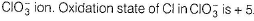QUESTION: 17

The products formed when slaked lime reacts with chlorine on heating

Solution: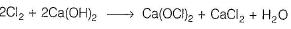QUESTION: 18

Which one among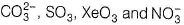have planar shape ?

Solution: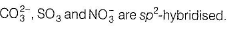QUESTION: 19

Which of the following molecules has a measurable dipole moment?

Solution:

BrF3 has ‘T’ shape while others have symmetrical shapes.

QUESTION: 20

When Cl2 gas reacts with hot and concentrated sodium hydroxide solution, the oxidation number of chlorine changes from

Solution: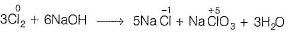QUESTION: 21

The geometry of the central atom in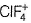is

Solution: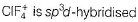with 4 bond pair and one lone pair.

QUESTION: 22

If A represents the central atom, in which molecule F-A-F angle is the smallest?

Solution: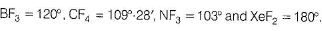QUESTION: 23

In the preparation of fluorine, electrolysis of molten KHF2 is preferred than molten fluorspar. The reason is

Solution:

Fluorine is prepared by electrolysis of fused KHF2, made as a mixture of KF(s)andHF(g)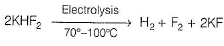QUESTION: 24

XeF6 on complete hydrolysis yields ‘X’. The molecular formula of X and its geometry respectively are

Solution: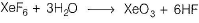XeO3 is a colourless explosive solid and has a pyramidal molecular structure.

QUESTION: 25

The ratio of the heat capacities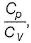for one mole of a gas is 1.6. The gas is

Solution: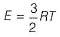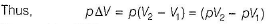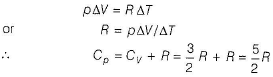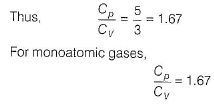QUESTION: 26

Which pair have identical shapes ?

Solution:

Both have linear shape.

QUESTION: 27

Which classification is correct?

Solution: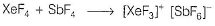QUESTION: 28

Which of the following is the life saving mixture of asthma patient?

Solution:

The mixture of 80% helium and 20% oxygen is used to arrest breathing in asthma and other respiratory diseases because helium is light and is less soluble in blood under high pressure.

QUESTION: 29

Match the shape to the formula, Which pairing is incorrect?

Solution:

XeO3 is pyramidal with sp3-hybridisation and others are correct key.

QUESTION: 30

The amount of Na2S2O3.5H2O required to completely reduce 100 mL of 0.25 N iodine solution is

Solution:

Milliequivaients of hypo = milliequivalents of iodine = 100 x 0.25
Gram equivalents of hypo = 0.025
Number of moles of hypo = number of milliequivalents of hypo = 0.025
Weight of hypo = 0.025 x 248 = 6.2 g